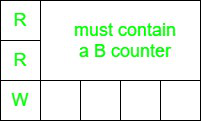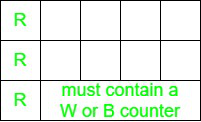GFG App
Open AppBrowser
Continue

# Puzzle | The Counters and Rectangular Board

Given 3N counters where N >= 1, there are three types of counters Red, White, and Blue all of them equal in count i.e., N and a rectangular board with 3 rows and N columns are filled with counters, the task is to rearrange the counters such that each column has counters of three different colors. The only operation allowed is to swap the counters in the same row. Design an algorithm to accomplish this task or prove that such an algorithm does not exist.

Solution:

1. For N = 1: The problem is trivial as all the 3 counters of a column are of different colors.
2. For N > 1: The problem can be solved by arranging the counters of 3 different colors for the first column and then, solving for the smaller instance of the problem. Consider the first column. There are three possibilities:
• All three counters are of different colors: In this case, nothing needs to be done.
• Exactly two counters are of the same color: Let’s assume that the two counters of the same color are red, and they are in the first two rows, whereas the counter in the first row, the third column is white, as shown. Since there must be N blue counters on the board, and no more than N-1 of them can be in the third row, this implies that at least one counter of blue color must be in the first two rows. Hence, the required algorithm can scan the first two rows, starting from the second column until a blue column is encountered; after a blue counter is found, it should be swapped with the counter in the first column.• All three counters are of the same colors: Let’s assume, that the three counters in the first column are red. This implies that each of the three rows now must now contain a at least one counter of a color other than the red, we can, therefore, scan any row, to obtain a counter of the different color and swap it with the counter in the first column to get the situation mentioned above.My Personal Notes arrow_drop_up